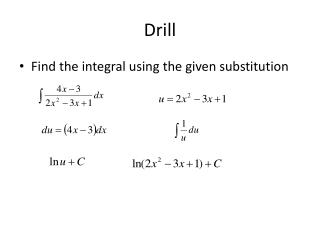DownloadDownload PresentationDrill

# Drill

Download Presentation## Drill

- - - - - - - - - - - - - - - - - - - - - - - - - - - E N D - - - - - - - - - - - - - - - - - - - - - - - - - - -
##### Presentation Transcript

1. Drill • Find the integral using the given substitution

2. Lesson 6.5: Logistic Growth Day #1: P. 369: 1-17 (odd) Day #2: P. 369/70: 19-29; odd, 37

3. Partial Fraction Decomposition with Distinct Linear Denominators • If f(x) = P(x)/Q(x) where P and Q are polynomials with the degree of P less than the degree of Q, and if Q(x) can be written as a product of distinct linear factors, then f(x) can be written as a sum of rational functions with distinct linear denominators.

4. Example: Finding a Partial Fraction Decomposition • Write the function f(x)= (x-13)/(2x2 -7x+3) as a sum of rational functions with linear denominators.

5. Write the function f(x)= (2x+16)/(x2 +x-6) as a sum of rational functions with linear denominators.

6. Example: Antidifferentiating with Partial Fractions Because the numerator’s degree is larger than the denominator’s degree, we will need to use polynomial division. 3x2 +3 - 3x4 -3x2 - 3x2 +1 3x2 -3 4

7. When x = -1 A(0) + B(-2) = 4; B = - 2 When x = 1 A(2) + B(0) = 4; A= 2

8. Finding Three Partial Fractions When x = -2 B(-4)(-3)=36 12B = 36 B = 3 When x = 1 C(-1)(3)=-6 -3C = -6 C = 2 When x = 2 A(4)(1)=4 4A=4 A=1

9. Drill: Evaluate the Integral When x = -7, B(-7) = -21, B = 3 When x = 0, 7A = 14, A = 2

10. The Logistic Differential Equation Remember that exponential growth can be modeled by dP/dt = kP, for some k >0 If we want the growth rate to approach 0 as P approaches a maximal carrying capacity M, we can introduce a limiting factor of M – P. Logistic Differential Equation dP/dt = kP(M-P)

11. General Logistic Formula • The solution of the general logistic differential equation is Where A is a constant determined by an appropriate initial condition. The carrying capacity M and the growth constant k are positive constants.

12. Example: Using Logistic Regression • The population of Aurora, CO for selected years between 1950 and 2003

13. Use logistic regression to find a model. • In order to this with a table, enter the data into the calculator (L1 = year, L2 = population) • Stat, Calc, B: Logistic • Based on the regression equation, what will the Aurora population approach in the long run? • 316,441 people (note that the carrying capacity is the numerator of the equation!)

14. When will the population first exceed 300,000? Write a logistic equation in the form of dP/dt = kP(M-P) that models the data. We know that M = 316440.7 and Mk =.1026 Therefore 316440.7k=.1026, making k = 3.24 X 10-7 dP/dt= 3.24 X 10-7 P(316440.7-P) • Use the calculator to intersect. • When x = 59.12 or in the 59th year: 1950 + 59 = 2009

15. 6.5, day #2 • Review the following notes, ON YOUR OWN • You can get extra credit if you complete the homework for day 2. (Will replace a missing homework, OR if you do have no missing assignments, it will push your homework grade over 100%) • Correct answers + work will give you extra credit on Friday’s quiz. • No work = No extra credit

16. Example • The growth rate of a population P of bears in a newly established wildlife preserve is modeled by the differential equation dP/dt = .008P(100-P), where t is measured in years. • What is the carrying capacity for bears in this wildlife preserve? Remember that M is the carrying capacity, and according to the equation, M = 100 bears

17. What is the bear population when the population is growing the fastest? The growth rate is always maximized when the population reaches half the carrying capacity. In this case, it is 50 bears. • What is the rate of change of population when it is growing the fastest? dP/dt = .008P(100-P) dP/dt = .008(50)(100-50) dP/dt =20 bears per year

18. Example • In 1985 and 1987, the Michigan Department of Natural Resources airlifted 61 moose from Algonquin Park, Ontario to Marquette County in the Upper Peninsula. It was originally hoped that the population P would reach carrying capacity in about 25 years with a growth rate of dP/dt = .0003P(1000-P) • What is the carrying capacity? 1000 moose Generate a slope field. The window should be x: [0, 25] and y: [0, 1000]

19. We first need to separate the variables. Then we will integrate one side by using partial fractions. • Solve the differential equation with the initial condition P(0) = 61

20. Multiply both sides by 1000 to clear decimals. Multiply both sides by -1 to put 1000-P on the top

21. Solve the differential equation with the initial condition P(0) = 61 Remember that ln(x) =y Can be rewritten as ey = x

22. Solve for P